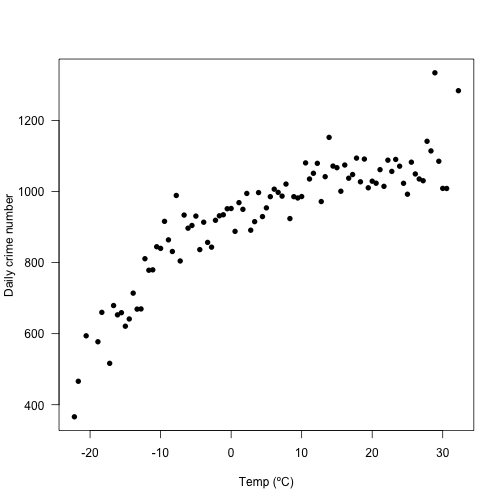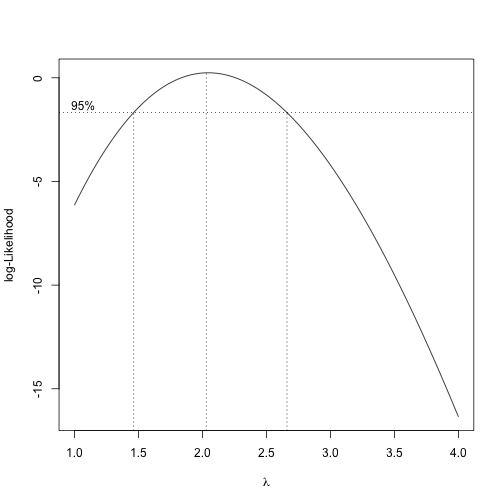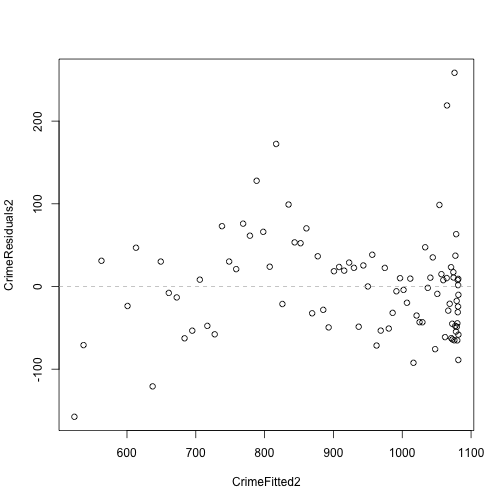# Exercise 4: CONTINUED - improving models

## Instructions:

Hints and reminders are italic

Questions appear in blue.

Needs to be completed and handed in by 21st February

The colleague that gave you the extra data has come back to see how you are getting on. They suggest that the main assumption not being met is linearity. A straight line does not seem to capture the data because it is curved. There are also some outliers.

Your team decides to remove the outliers. You have reason to believe they might be typos.

9. What are some positives and negatives of removing outliers? What things should you consider when removing them?

``````# Remove the outliers (my data was called WithFreeze)
# This is done by using indexing brackets [,]
# These work by searching inside a data set
# by row first then column e.g. [row,column]
# Here we take ALL columns, which is why it is blank.
# But we only take rows which have a residual of
# less than 200. Basically we move the data that
# created the highest residuals

NoOutliers <- WithFreeze[residuals(model2) < 200,]

plot(NoOutliers\$TempC, NoOutliers\$Crime, pch=16, xlab="Temp (ºC)",
ylab="Daily crime number", las=1)
``````The data is still curved. So you will want need to use a transformation of the response variable or a polynomial (square or cube etc). But which one?

You can use Box-Cox to indicate what kind of transformation might help with improve the linear regression. The plot shows the likelihood for different powers of transformation. E.g. 2 is a squared transformation, 3 is cubic etc.

``````# You might need to install the package MASS
# install.packages("MASS")

# Run the
MASS::boxcox(model2, lambda = seq(1,4, length=30))
``````Box-Cox suggests that a quadratic (x2) transformation. You could either transform the response variable OR add a quadratic term as an explanatory variable. You choose to try the second and add the quadratic term.

``````# Create a linear model
# to add a quradtic (or any power) term you must right the
# explanatory variable as I(variable^2) AND keep in the original
# explanatory variable. See example below.
# We need both the linear and quadratic components.

model3 <- lm(Crime ~ TempC + I(TempC^2), data = NoOutliers)

# Also plot the Residuals vs fitted
# create a vector of rounded residuals
CrimeResiduals2 <- round(residuals(model3),2)

# create a vector of rounded fit
CrimeFitted2 <- round(fitted(model3),2)

# plot the fitted and residuals
plot(CrimeFitted2, CrimeResiduals2)
# add a horizontal line at 0
# line is grey and dashed (lty=2)
abline(h=0, lty=2, col="grey")
``````9. Look at the new Residuals vs Fitted plot. What do you think of this new model? Has it improved the fit?

10. What about usefulness? How much of the variance in daily crime numbers is this model explaining? Work out using R squared and explain what this measures

Hint(basically the whole code): summary(model)\$r.squared

11. Now predict again from the new model. Does this change your recommendation for the number of police needed? If so, how?

12. Think about the biological context of the results. Why could there be a quadratic relationship between daily crime numbers and temperature? How could you try to find out what the reasons are? E.g. new studies or data you would need